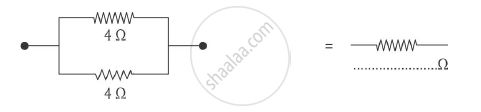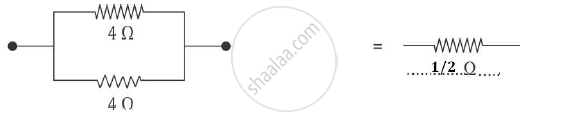# Complete the Following - Science and Technology 1

Complete the following :-

(b)#### Solution

(b)"The equivalent resistance is "R_p=1/4+1/4=(1+1)/4=1/2Omega

Concept: System of Resistors - Resistances in Parallel
Is there an error in this question or solution?
2014-2015 (March)

Share